# Powers: Simplifying Expressions with Exponents

• Introduction

• Properties of exponentials

• Solved Exercises: Simplifying Expressions with Exponents

## Introduction

Power is an expression of this type

## ab = a · a · · · a · a

that represents the result of multiplying the base, a, by itself as many times as the exponent, b, indicates. We read it as "a to the power of b".

For example, 2 3 = 2·2·2 = 8 (the base is 2 and the exponent is 3).

Generally, the base as well as the exponent can be any number (real or complex) or they can even be a variable, unknown factor or parameter. The equations with the unknown factor is in the exponent are known as exponential equations.

A special case are powers whose exponents are fractions. In this case, the power represents a square root. They appear due to the need to solve an equation of the type x n = a.

Another special case are powers with a base of 10, ones with this appearance 10 n. If n is a natural number (0, 1, 2, 3,...) the result is 10...0, being n the number of 0's. If n is a negative number (-1, -2, -3, -4,...), the result is 0.00...1 where the value of n in positive indicates the number of 0's counting the 0 before the comma. These are the type of powers used in scientific notation.

Finally, we'll say that the power elevated to 0 is always 1, so, x 0 = 1.

In this section, the activities are in order of increasing difficulty: we use the properties of exponentials (power of products, power of quotient, power of a power,..) and, after we'll simplify expressions formed by powers (parenthesis, fractions, negative exponents, parameters..).

### PROPERTIES OF POWERS

Product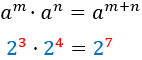Power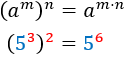Quotient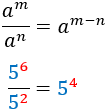Negative exponent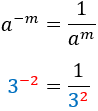Inverse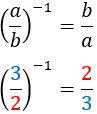Inverse of inverse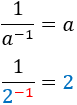# Solved Activities

Exercise 1Show solution

Exercise 2Show solution

Exercise 3Show solution

Exercise 4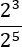Show solution

Exercise 5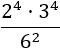Show solution

Exercise 6Show solution

Exercise 7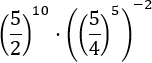Show solution

Exercise 8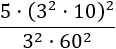Show solution

Exercise 9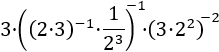Show solution

Exercise 10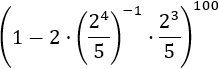Show solution

Exercise 11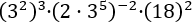Show solution

Exercise 12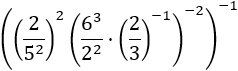Show solution

Exercise 13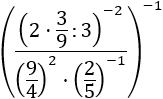Show solution

Exercise 14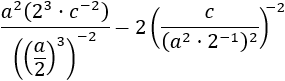Show solution

Exercise 15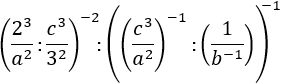Show solution